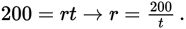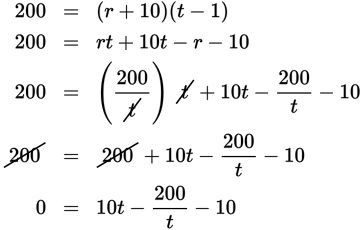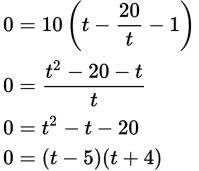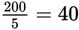# SAT Math Multiple Choice Question 473: Answer and Explanation

### Test Information

Question: 473

8. A freight train operator knows that on a 200-mile trip, if the freight cars are not fully loaded, she can save 1 hour of travel time by increasing her normal speed by 10 miles per hour. What is her normal speed in miles per hour?

• A. 40
• B. 45
• C. 55
• D. 60

Explanation:

A

Difficulty: Medium

Category: Problem Solving and Data Analysis / Rates, Ratios, Proportions, and Percentages

Strategic Advice: When a question concerns distance, rate, and time, organizing the information in a table is usually very helpful.

Getting to the Answer: Use the equation Distance = rate × time.

 Distance Rate Time Normal Trip 200 r t Faster Trip 200 r + 10 t – 1

Now, set up a system of equations: 200 = rt and 200 = (r + 10)(t – 1). Solve the system by solving the first equation for r and substituting the result into the second equation; the first equation isTip: Go ahead and FOIL the factors in the second equation before substituting the value of r.Now, factor out a 10, find a common denominator, and you'll wind up with a fairly nice quadratic equation to solve.The two solutions are t = 5 and t = –4. Time can't be negative, so t = 5. Substitute this back into the first equation (because the question asks about her original speed) and solve for r. Her normal speed ismiles per hour.